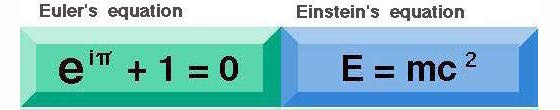﻿ E

Swiss born Leonhard Euler (1707-1783) was the most prolific mathematician of all time and he did for modern analytic geometry and trigonometry what the 'Elements' of Euclid had done for ancient geometry.
He found the most remarkable equation in all of mathematics, which involves the number  "e".

### e  -  a most mysterious number in mathematics

From computer science to statistics, the number  e  is everywhere in the mathematical sciences.
The notion of   "e"  was first introduced by Leonhard Euler in 1731.

e  is the symbol for the base of natural logarithms. Along with  pi,  e  has transformed our understanding of the very concept of number. Far from being invented by mathematicians, both numbers exist in their own right and crop up throughout the natural world.  e  plays a key role in describing of how radioactive decay progresses; in the underlying processes of slide rules, etc.

e and  pi are both examples of  transcendental numbers, a type of number whose baffling complexity is the very antithesis of the plain everyday  integers  0, 1, 2, 3, 4  and so on. Whereas the integers are easy for humans to understand, transcendental numbers are infinitely harder to pin down.
All transcendental numbers are irrational numbers. But the converse is not true; there are some irrational numbers that are not transcendental.

 Definition of a transcendental number e and  pi are examples of special irrationals known as transcendental numbers. A transcendental number is entirely unrelated to the integers by any sequence of ordinary arithmetical operations. You can multiply it by itself as many times as you wish, combine these powers and divide and multiply by integres in whatever fashion you want, but you will never arrive back in the familiar territory of the integers. This is the definition of a transcendental number. Even numbers like the square root of 2 are tame compared with transcendentals. By definition if you multiply the square root of 2 by itself the result is  2  -  an ordinary number, or an integer. So we get back to the integers after just one step.   But  e  and  pi  are different, no ordinary calculation will connect them back to ordinary numbers. It was the genius of the great swiss mathematician Leonhard Euler to find a way to tie several transcendental numbers into a single equation as shown below. For mathematicians Euler's equation became as important as the more famous equation of Einstein is for physicists.In one awe-inspiring equation Euler tied  e  to the four other fundamental numerical entities  0,  1,  pi,  and  i.   e  as the symbol for the base of natural logarithms;  i  for the square root of  -1, and  pi  for the ratio of circumference to diameter in a circle  -  all three transcendental numbers (that is non-terminating) which means they are not precisely defined, but - almost magically - in Euler's equation they unite as a whole precisely defined ! Euler's formula became central to our understanding of number and exponentiation, and is celebrated for the beautiful way it unites the five fundamental constants of mathematics. After demonstrating a proof of this equation a famous mathematician told the audience: "Gentlemen it is absolutely paradoxical; we cannot understand it, and we don't know what it means. But we have proved it, and therefore we know it is the thruth." The physicist Richard Feynman described it as "the most remarkable formula in mathematics" . What an incredible achievement : to mathematically proof something that we cannot yet understand ! For more explanation go to :  The number  e   The decimal expansion for e begins: e = 2.718281828459......

 ( The above text is excerpted from the NewScientist, July 21-27, 2007 )